# Sequences

### Term 1 starting in week 1 :: Estimated time: 2 weeks

• Describe and continue a sequence given diagrammatically
• Predict and check the next term(s) of a sequence
• Represent sequences in tabular and graphical forms
• Recognise the difference between linear and non-linear sequences
• Continue numerical linear sequences
• Continue numerical non-linear sequences
• Explain the term-to-term rule of numerical sequences in words

For higher-attaining pupils:

• Find missing numbers within sequences
• Finding the nth term of a sequence

This page should remember your ticks from one visit to the next for a period of time. It does this by using Local Storage so the information is saved only on the computer you are working on right now.

## Lesson Starters

Here are some suggestions for whole-class, projectable resources which can be used at the beginnings of each lesson in this block.

### 1st Lesson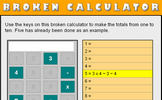#### Broken Calculator (3 and 4)

Some keys are missing from this calculator. Just how useful is it?

### 2nd Lesson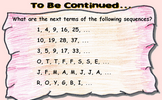#### To Be Continued

Work out the next term in the given sequences.

### 3rd Lesson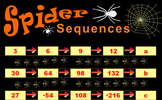#### Spider Sequences

Find the next term of the given number sequences. Can you also find a general rule for predicting the nth term of the sequence?

### 4th Lesson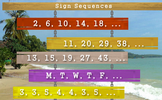#### Sign Sequences

Continue the sequences if you can work out the rule.

### 5th Lesson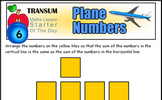#### Plane Numbers

Arrange numbers on the plane shaped grid to produce the given totals

### 6th Lesson#### Secret Symbol

Place a symbol between the four and the five to get a number greater than four and less than five.

Some of the Starters above are to reinforce concepts learnt, others are to introduce new ideas while others are on unrelated topics designed for retrieval practice or and opportunity to develop problem-solving skills.

White Rose ResourcesEnd of block assessments provide a quick progress check at the end of each block of learning to make sure students have understood the content covered. This Scheme of Learning was produced by White Rose Maths and is used here with permission granted on 30th June 2021.For All: